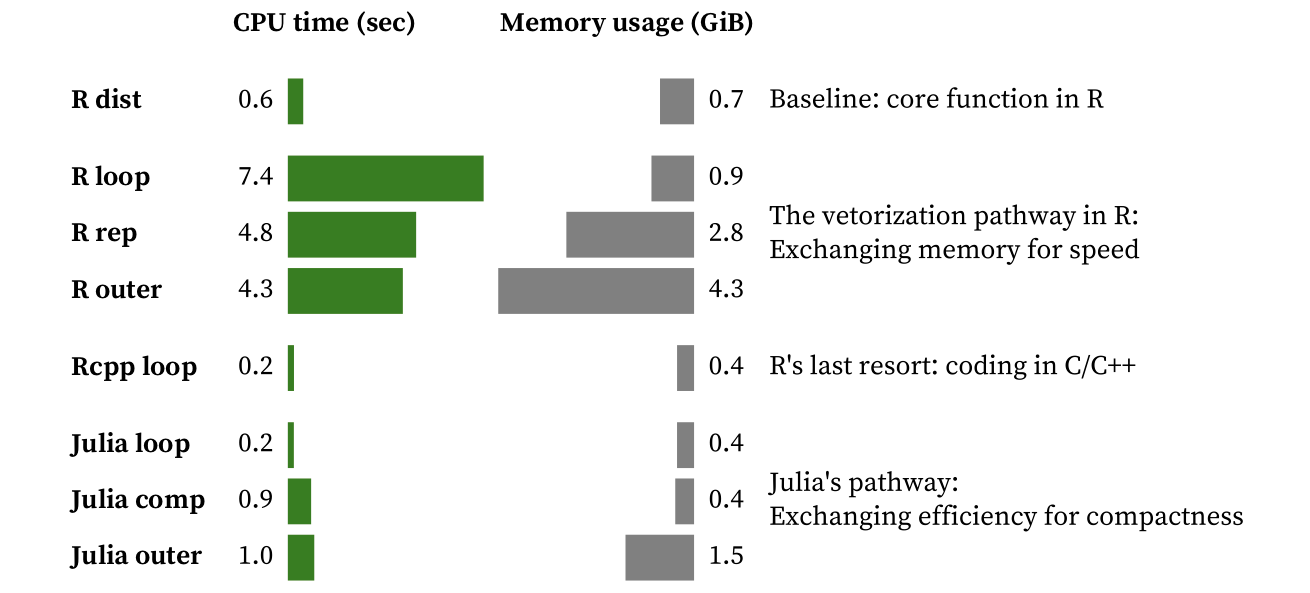.

# Julia vs R: Freeing the data scientist mind from the curse of vectoRization

Nowadays, most data scientists use either Python or R as their main programming language. That was also my case until I met Julia earlier this year. Julia promises performance comparable to statically typed compiled languages (like C) while keeping the rapid development features of interpreted languages (like Python, R or Matlab). This performance is achieved by just-in-time (JIT) compilation. Instead of interpreting code, Julia compiles code in runtime. While JIT compilation has been around for sometime now (e.g. Matlab introduced it in 2002), Julia was designed for performance with JIT compilation in mind. Type stability and multiple-dispatch are key design concepts in Julia that put it apart from the competition. There is a very nice notebook by the Data Science Initiative at the University of California that explains these concepts if you want to learn more.

Somewhere in time, we started using interpreted languages for handling large datasets (I guess datasets grew bigger and bigger and we kept using the same tools). We learned that, for the sake of performance, we want to avoid loops and recursion. Instead, we want to use vectorized operations or specialized implementations that take data structures (e.g. arrays, dataframes) as input and handle them in a single call. We do this because in interpreted languages we pay an overhead for each time we execute an instruction. While I was happy coding in R, it involved having a set of strategies for avoiding loops and recursion and many times the effort was being directed to “how do I avoid the pitfalls of an interpreted language?”. I got to a point where I was coding C functions to tackle bottlenecks on my R scripts and, while performance clearly improved, the advantages of using R were getting lost in the way. That was when I started looking for alternatives and I found Julia.

Next, I will try to show you how Julia brings a new programming mindset to Data Scientists that is much less constrained by the language.

Experiments

Let us consider the problem of calculating the distances among all pairs of elements in a vector with 10.000 elements. A solution for this problem requires ~50M to 100M distance calculations (depending on the implementation). The following approaches were implemented and benchmarked:

• R dist: R's built-in function for solving our problem (baseline)
• R loop: nested loops approach in R (14 lines of code)
• R rep: loops replaced by R's rep function plus vectorized operations (6 lines of code)
• R outer: using R's outer product function plus vectorized operations (4 lines of code)
• Rcpp loop: C++ pre-compiled implementation based on nested loops integrated with R via the Rcpp package (17 lines of code)
• Julia loop: nested loop approach in Julia (15 lines of code)
• Julia comp: loops represented as arrays comprehensions in Julia (2 lines of code)
• Julia outer: direct translation of R outer approach to Julia (4 lines of code)

ResultsThe loop-based implementation in R was the slowest, as expected (and would be much slower before version 3.4 where JIT became available). By vectorizing, we decrease computation time but increase memory consumption, which can become a problem as the size of the input increases. Even after our vectorization efforts, we are still far from the performance of R’s dist function.

Rcpp allowed decreasing both computation time and memory requirements, outperforming R’s core implementation. This is not surprising as R’s dist function is much more flexible, adding several options and input validation. While it is great that we can inject C/C++ code into R scripts, now we are dealing with two programming languages and we have lost the goodies of interactive programming for the C++ code.

Julia stands out by delivering C-like performance out of the box. The tradeoff between code compactness and efficiency is very clear, with C-like code delivering C-like performance. Thus, the most efficient solution was based on loops and preallocating memory for the output. Comprehensions are a good compromise as they are simpler to code, less prone to bugs, and equally memory-efficient for this problem.

comprehensive version of this article that includes the code used for the experiments was originally published at here (open access)

Views: 20646

Comment